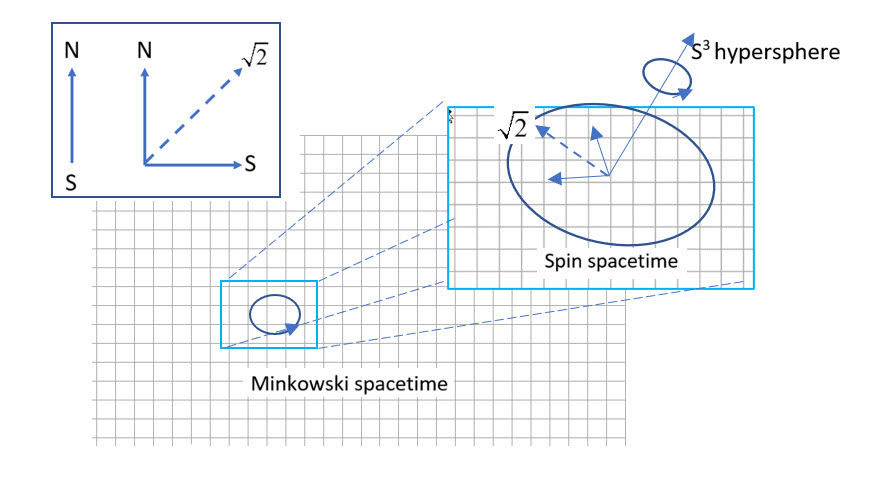Preprint Article Version 1 Preserved in Portico This version is not peer-reviewed

# Extrinsic Quaternion Spin

Version 1 : Received: 1 February 2023 / Approved: 3 February 2023 / Online: 3 February 2023 (03:51:33 CET)

How to cite: Sanctuary, B. Extrinsic Quaternion Spin. Preprints 2023, 2023020055. https://doi.org/10.20944/preprints202302.0055.v1. Sanctuary, B. Extrinsic Quaternion Spin. Preprints 2023, 2023020055. https://doi.org/10.20944/preprints202302.0055.v1.

## Abstract

We present an analysis of the Dirac equation when the spin symmetry is changed from SU(2) to the quaternion group, $Q_8$, afforded by multiplying one of the $\gamma$-matrices by the imaginary number $i$. The resulting spin equation separates into distinct and complementary Hermitian and antiHermitian spaces. The former describes a 2D structured spin and the latter its helicity, generated by a unit quaternion. We discuss some consequences.## Keywords

foundations of physics; Dirac equation; spin; quantum theory; non-locality; helicity

## Subject

PHYSICAL SCIENCES, General & Theoretical Physics

Comment 1
The commenter has declared there is no conflict of interests.
Comment:
• The paper makes some changes to the Dirac equation and then draws all sorts of conclusion, claiming they are a consequences. Mathematical words are used inappropriately and without apparent understanding throughout the paper. E. g.
• What is a hermitian space? The strange mathematics makes it difficult to discuss.

What we can say for sure is that a difference representation of the gamma matrices gives no new physics.

However using matrices that do not satisfy the Clifford algebra might seem like a small and acceptable change to the author, but it is not. The resulting equation simply won't work. It won't transform correctly under spacetime symmetries and you won't be able to define a conserved particle number.

It is not worth proceeding further into the detail of the paper when it is based on equations that cannot represent real particles.

If the author wants to change the Dirac equation he first needs to show in detail that 1) all important results are still valid 2) if it is valid then the next step is to show that it is physically different.
+ Respond to this comment
Response 1 to Comment 1
Commenter:
The commenter has declared there is no conflict of interests.
Comment: Mark Hadley comments are disingenuous. He fails to understand the presentation follows Dirac's seminal work, so if he says I am wrong, he is also saying Dirac is wrong.

The only change I made is to multiply one of the well known gamma matrices by the imaginary number "i". From that small change the rest follows.

In the last two points, Hadley ignores the fact that I show the resulting quaternion equation does indeed recover the original Dirac equation. The new result is quaternion spin which reveals properties that formulate coherence This is new and its implication appear to have far reaching consequences for our understanding of the foundation of physics

Views 0